# Texas Go Math Grade 2 Unit 4 Answer Key Geometry and Measurement

Refer to our Texas Go Math Grade 2 Answer Key Pdf to score good marks in the exams. Test yourself by practicing the problems from Texas Go Math Grade 2 Unit 4 Answer Key Geometry and Measurement.

## Texas Go Math Grade 2 Unit 4 Answer Key Geometry and Measurement

Show What You Know

Check your understanding of important skills

Order Length

Question 1.
Order from shortest to longest. Write 1, 2, 3.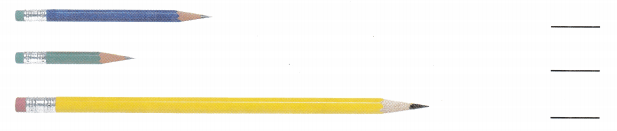Explanation:
I wrote the numbers for the according to their lengths from shortest to longest.The green pencil is shortest, the blue pencil is longerthan green pencil and the yellow pencil is longest pencil.

Time to the Hour

Write the time shown on the clock.

Question 2.Explanation:
The time the clock shows is 11:00.

Texas Go Math Grade 2 Geometry Unit 4 Answer Key Question 3.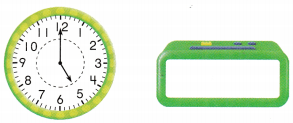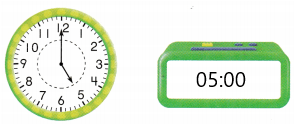Explanation:
The time the clock shows is 05:00.

Identify Two-Dimensional Shapes

Question 4.
Draw a loop around the rectangles. Draw an X on the triangles.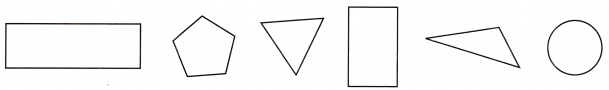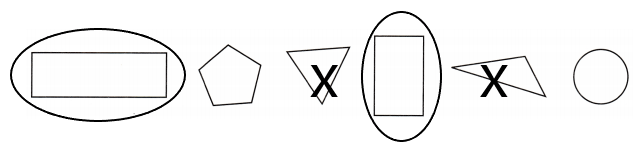Explanation:
I circled the rectangles and marked X on the triangles.

FAMILY NOTE: This page checks your child’s understanding of important skills needed for success in Unit 4.

Vocabulary Builder

Visualize It

Fill in the graphic organizer to describe the lengths of different objects.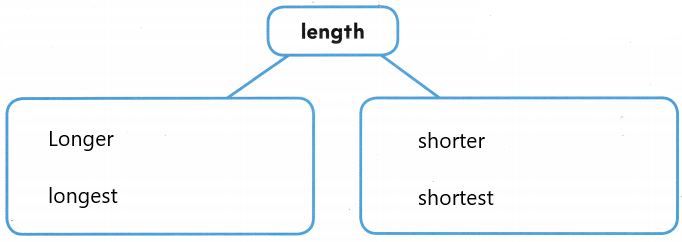Understand Vocabulary

Use review words. Complete the sentences.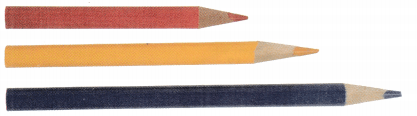Review Words
length
longer
shorter
longest
shortest

Question 1.
The blue pencil is the __________________ pencil.
The blue pencil is the longest pencil.

Question 2.
The red pencil is the ___________________ pencil.
The red pencil is the shortest pencil.

Question 3.
The red pencil is __________________ than the yellow pencil.
The red pencil is shorter than the yellow pencil.

Texas Go Math Geometry Grade 2 Unit 4 Test Answer Key Question 4.
The blue pencil is _________________ than the yellow pencil.
The blue pencil is longer than the yellow pencil.

Figures
written by Mary Gobles
This Take-Home Book belongs to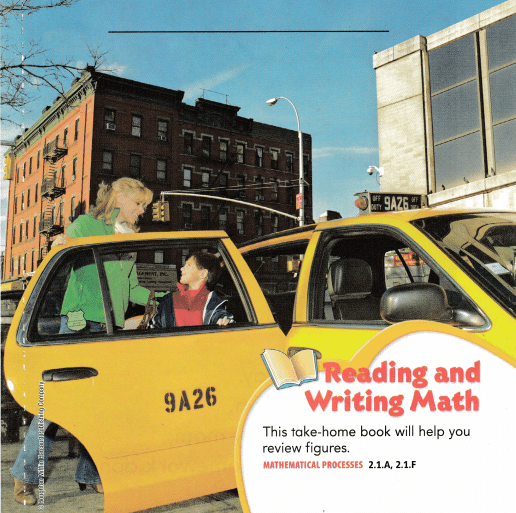We’re on our way. It is sure to be fun. But I promised my mom, I’ll get my math homework done.What do the homework directions say to do?I found the first figure, it has sides 1, 2, 3. Yes, it’s a triangle above a door. Circle the figure, then you can find A figure with sides 1, 2, 3, 4.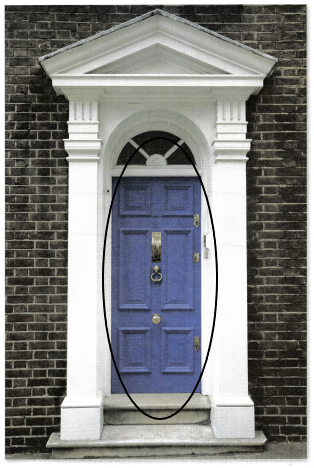Explanation:
I circled the door as it is in the shape of rectangle.As we rode along, I saw from the cab A very tall building we’d pass.
I looked at its windows, each one the same shape.
They were squares that were made out of glass!
Circle a square.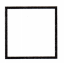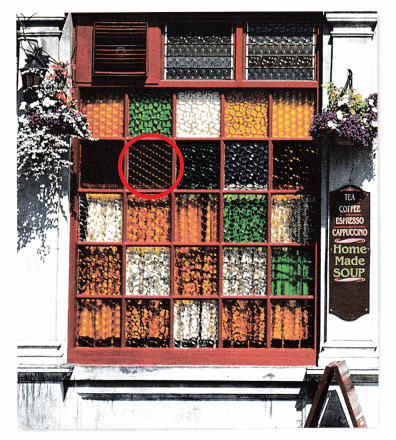Explanation:
I circled one of the window a sit is in the shape of square.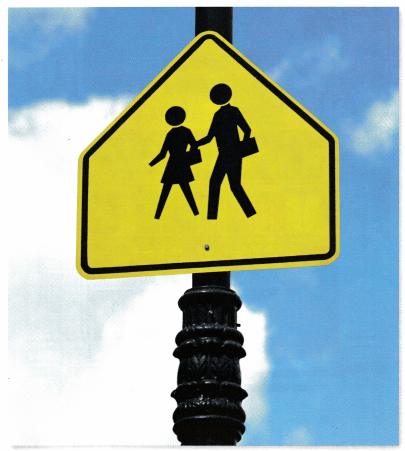A 5-sided figure was hard to find But there on the lightpost, I could see A pentagon right in the middle.
Circle the figure for me.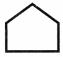Explanation:
I circled the traffic sign board as ot is in the shape of pentagon.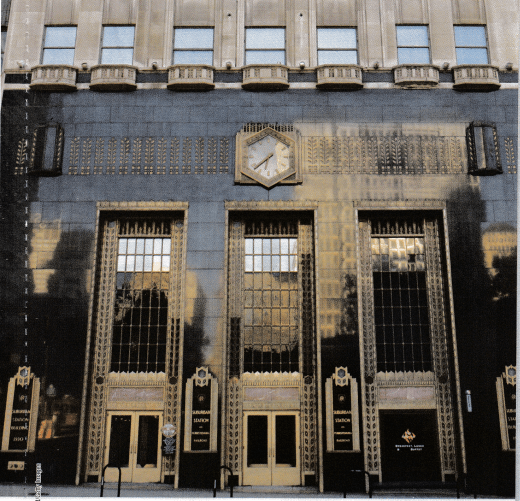The last figure to find is common.
The rectangle is the figure’s name.
When I found it I shouted, ‘Hip, hip hurrah!
Mom, this was such a fun game.”
Circle a rectangle.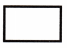Explanation:
the large window is in the shape of a rectangle.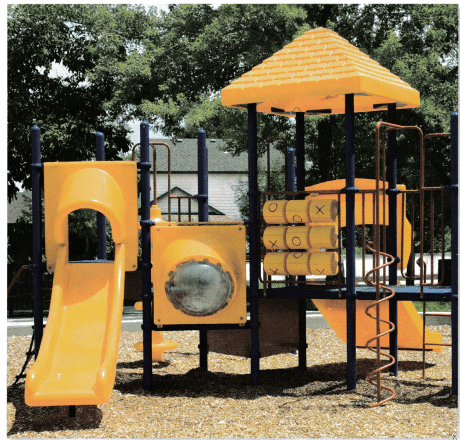We got to the park, and I could play. My math homework was all done! I liked finding figures, and so I continued To find figures while having some fun!
What figures do you see in the park?
In the park there are different shapes, they are rectangle, square, triangle and circle.

Write Math Look at the shapes. Draw a picture that has these shapes in it. Write a story about your picture.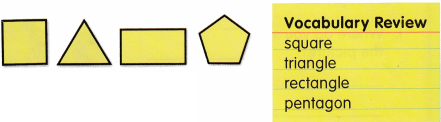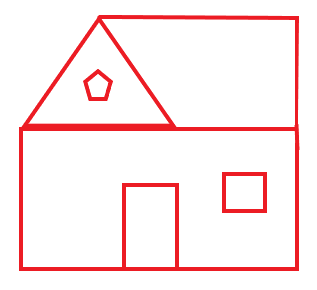Explanation:
John has a house made of different. The door in the shape of a rectangle, the window in the shape of square, the top of the house in the shape of traingle and a pentagon at the top.

Describing Shapes

Use the picture to answer the questions.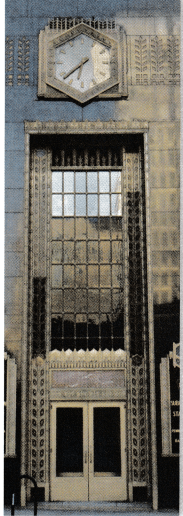Question 1.
Where do you see rectangles in the picture?
Doors and windows are in the shape of rectangles.

Question 2.
How many sides does one rectangle have?
_______ sides
A rectangle has 4 sides.

Unit 4 Test Congruent Triangles Answer Key Pdf Question 3.
Look at the large window above the doors. How many small rectangles are in the first three rows of the rectangles?
_____________ small rectangles
There are 18 small rectangles in the first three rows of the rectangles.

Question 4.
Look at the face of the clock. How many sides does it have?
________ sides